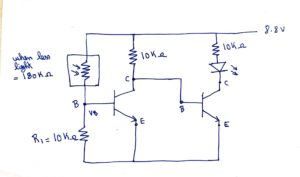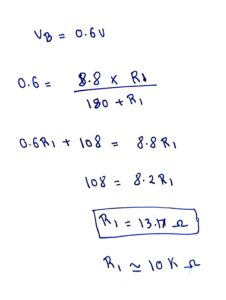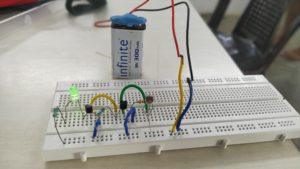# Automatic street light using LDR

~Ranjith, Abilash

While preparing for the electronics class. we took “Automatic street light using LDR” as the circuit, where “LED” turns ON in dark, and turns OFF in bright place.

After giving the circuit connection, the LED didn’t turn OFF when it was bright. So we started analyzing the circuit, then we found out that, the R1 resistor was low when compared to the resistance of the LDR under the brightness.

We calculated the resistor value of R1, by assuming that

1. Vbe = 0.6v was needed to turn ON the “transistor 1”.
2. Resistance of LDR = 180 kΩ under minimum light for which LED should be OFF (if we further reduced the light, resistance will increase, then “LED” should turn ON and “transistor 1” should turns OFF).

From the calculation, we got that R1 = 13.17 kΩ, we approximate it to 10 kΩ.Finally we made it to work.